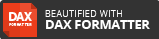cancel
Showing results for
Did you mean:## Power BI Desktop/DAX – Using Unicode (Arrows) as Measures in a Table

I was helping out in the Power BI Community and I came across the following requirement.

The user had sales figures and based on the Previous month, they wanted to have an arrow showing if it was up or down. As well as if there was no data to say that there was no data. So this is how I achieved this below.## Creating a Date Table to make PREVIOUSMONTH DAX measure easy

The first thing that I did was to create a Date table and link this to my table above.

This ensured that when I wanted to create my PREVIOUSMONTH DAX measure it would be simple and easy.

I did it using my blog post: Power BI – How to Easily Create Dynamic Date Table/Dimension with Fiscal Attributes using Power Quer...

Once I had my Date Table I then created the relationship between my Sales Data table and my Date Table## Creating PREVIOUSMONTH DAX measure

Next I created my Previous Month measure with the following DAX Syntax

Previous Month Sales =

CALCULATE ( [Sales Amount], PREVIOUSMONTH ( 'Date'[Calendar Date] ) )## Getting the Unicode numbers for Arrows

Now in order to get the Unicode Characters I followed the very interesting blog post by Chris Webb: The DAX Unichar() Function And How To Use It In Measures For Data Visualisation, in which I learnt how to use Unicode Characters in a measure.

Next I found the following website which contained the Unicode numbers for my arrows I required.

https://unicode-table.com/en/sets/arrows-symbols/

I then made a note of the following ones that I wanted to use## Creating the Arrows measure

The final piece was where I created the Arrows measure.

Below is the DAX Syntax with an explanation afterwards

Arrows = SWITCH(

TRUE(),

ISBLANK([Previous Month Sales]),BLANK(),

|| ISBLANK([Sales Amount]),BLANK(),

[Sales Amount] <= 0, "No Data",

[Sales Amount] >= [Previous Month Sales],UNICHAR(9650),
[Sales Amount] <= [Previous Month Sales],UNICHAR(9660),

BLANK()

)• Due to having multiple conditions instead of having nested IF statements I found it easier to use the SWITCH DAX function.
• I also know that with the SWITCH DAX function it evaluates from top to bottom.
• So the first condition was to check for if the [Previous Month Sales] or the [Sales Amount] was blank meaning it was the start or end of the data to make it BLANK. It is highlighted in GREEN
• This could be changed to anything value, but I prefer it being BLANK.
• Next I put in a condition to see if the current Months [Sales Amount] is less than or equal to 0 to put in the value of “No Data” It is highlighted in PURPLE
• Next is where I use the Unicode characters in my measure. Here I compare if the Sales for the [Sales Amount] is >= the [Previous Month Sales]and if it is then use the UP Arrow. This is highlighted in BLUE.
• Next is where I use the Unicode characters in my measure. Here I compare if the Sales for the [Sales Amount] is <= the [Previous Month Sales], and if it is then use the DOWN Arrow. This is highlighted in ORANGE.
• And then finally if none of the above conditions are met then make the value BLANK

## Table Output

Below is the outputted table, which is doing as what was required.## Conclusion

As you can see from above being able to make sure of the Unicode Characters can make your dataset that much easier to read.

You can view it here: PBI – Unicode Measures